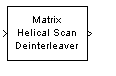# Matrix Helical Scan Deinterleaver

Restore ordering of input symbols by filling matrix along diagonals

## Library

Block sublibrary of Interleaving

•## Description

The Matrix Helical Scan Deinterleaver block performs block deinterleaving by filling a matrix with the input symbols in a helical fashion and then sending the matrix contents to the output port row by row. The Number of rows and Number of columns parameters are the dimensions of the matrix that the block uses internally for its computations.

Helical fashion means that the block places input symbols along diagonals of the matrix. The number of elements in each diagonal matches the Number of columns parameter, after the block wraps past the edges of the matrix when necessary. The block traverses diagonals so that the row index and column index both increase. Each diagonal after the first one begins one row below the first element of the previous diagonal.

The Array step size parameter is the slope of each diagonal, that is, the amount by which the row index increases as the column index increases by one. This parameter must be an integer between zero and the Number of rows parameter. If the Array step size parameter is zero, then the block does not deinterleave and the output is the same as the input.

This block accepts a column vector input signal. The number of elements of the input vector must be the product of Number of rows and Number of columns.

The block accepts the following data types: `int8`, `uint8`, `int16`, `uint16`, `int32`, `uint32`, `boolean`, `single`, `double`, and fixed-point. The output signal inherits its data type from the input signal.

## Parameters

Number of rows

The number of rows in the matrix that the block uses for its computations.

Number of columns

The number of columns in the matrix that the block uses for its computations.

Array step size

The slope of the diagonals that the block writes.

## Pair Block

Matrix Helical Scan Interleaver